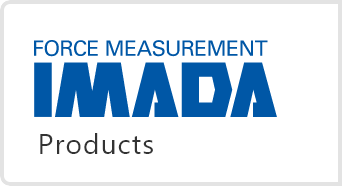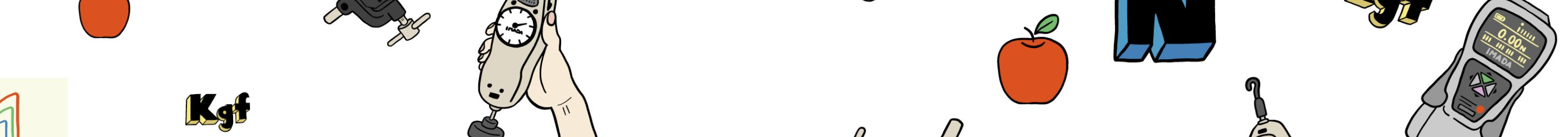# Coffee break## Introduction to Friction Testing (1) Surface-to-Surface Contact Friction Testing

Nov 01,2023

1) Friction Test for measuring slipperiness and non-slipperiness

2) Frictional Force and Coefficient of Friction

3) Friction Test for Printed Paper

4) Summary

## 1) Friction test to measure slipperiness and slip resistance

Do you know the details of a Friction test?

It is a test to measure the frictional force (frictional resistance) = the force generated when two objects in contact are set to slide. This test is used for various purposes, such as “How much force must be applied to move an object?”, “How much force can an object withstand at rest without moving?”, etc.

The following test is an example of a friction test performed by a company or research institute.

– Printed paper quality test

– Operability measurement for the smartphone display protection film

– Writing comfort test of writing instruments

– Quality testing of lubricants and greases

In this article, we are introducing “Friction testing of printed papers” as an example of testing frictional forces of surface-to-surface contacts of two objects. Paper friction tests are essential in many industries, especially paper and packaging.

You might also have heard the wear tests, similar to the friction tests. But as the word wear, it means wearing, grinding, or rubbing away by friction. Wear test is a test to observe the destruction of objects. The surface condition and the mass lost due to shedding are analyzed. Friction and wear tests are sometimes performed independently, but they are often proceeded simultaneously due to their functions.

## 2) Frictional Force and Coefficient of Friction

Before getting into the details, let us review the basics. As mentioned earlier, friction is a phenomenon in which a force (frictional force) is generated to prevent the movement of two objects in contact when moving in a sliding motion. The magnitude of the Frictional Force is the same as “the magnitude of the force applied to move the object” when the object is at rest. Still, its maximum valueis affected by “the nature of the object’s resistance to sliding” and “the force applied to the contacting object from the contacting surface (Normal force).” The object remains in place until a force exceeding the maximum frictional force is applied, and the object begins to move when an applied force exceeds the maximum frictional force.

*Normal force refers to the resistance force acting perpendicular to the object from the contact surface. When an object applies a force to a contact surface, a force of the same magnitude acts on the side of the object to which applies the force. If an object is placed on a horizontal surface, the normal force is the value obtained by converting the object’s mass into a force (gravity).

In the world of physics, the following relationship holds.

Maximum Frictional Force (N) = Normal Force (N) x Coefficient of the object’s resistance to slippage

“Coefficient of the object’s resistance to slippage” is called Coefficient of Friction, which can be calculated by the following equation.

Coefficient of Friction = Maximum Frictional Force (N) / Normal Force (N)

For example, if an object weighing 200g is placed on a horizontal table and the force when the object is pushed horizontally and starts moving is 1.5N, the coefficient of (static) friction = 1.5/1.96133 = 0.765 (*calculated at 1kgf = 9.80665N). By calculating the coefficient of friction, it is possible to eliminate the influence of the sample mass and compare the property of slip resistance.

However, frictional force is affected by test speed, contact area, and test environment (temperature, humidity, etc.), so when comparing coefficients of friction, it is essential to compare the results of tests with the same conditions. It is also important to note that the coefficient of friction is a coefficient for two objects in contact, so it should be considered the coefficient of friction between object A and object B. In addition, the coefficient of friction is affected by the surface condition, so the value is not necessarily constant.

## 3) Friction Test of Printing Paper

So here is the actual example of the friction test the manufacturers are conducting: the Testing Printing Paper Friction. Some of you may not be familiar with paper friction tests. These tests are conducted to check paper’s physical properties and characteristics, such as “impact on paper feeding during printing” and “resistance to collapse when placed in stacks” etc. JIS P 8147; 2010(Paper and board – Determination of the static and kinetic coefficients of friction) is one of the standards for paper friction testing. In the standard, the frictional force and coefficient of friction are measured by placing a weight on the top of 2pcs of printing paper, and one on top is pulled to slide to create the frictional force.

The graph above shows the result of an actual friction test of 2 pcs of printing papers. The vertical axis is the frictional force (N), and the horizontal axis is the time (seconds).

The large frictional force is identified at the beginning of the movement. Normally, the highest frictional force is generated before the object moves from a stationary state with a force application. This frictional force is called the Static Frictional Force (SFF). On the other hand, the frictional force decreases slightly after the object begins to move, and the curve remains stable. It means the maximum frictional force differs between static and moving. The frictional force during motion is called the Kinetic Frictional Force KFF.

The results by dividing the static and kinetic frictional forces using the normal force (calculated from the weight mass) are called the Static and Kinetic Friction Coefficients, respectively. The coefficient of kinetic friction is often calculated from the average kinetic frictional force within a certain range of motion and is analyzed using graphing software.

Precautions to be taken during testing include “Do not repeat measurements on the same sample,”

The test results for repeated measurements on the same sample are invalid except for intentional cases. This is due to the surface condition changes of the sample specimen, affecting the measurement even if no apparent changes are identified.

For example, the graph and comparison chart below display the results of multiple Friction tests on sandpaper and plywood using the same sample repeatably, showing the decrease in the average dynamic coefficient Friction after the first, fifth, and tenth tests.

Although sandpaper is an obvious example, this would be the case with all samples to varying degrees (Some samples have a higher coefficient of friction with repetition). Additionally, sample surfaces must be kept untouched, to avoid sebum from the hand which may act as a lubricant and affect the frictional force. In addition, as mentioned in Section 2, it is important that comparisons are made between test results with the same test conditions.

## 4) Summary

In this article, we have introduced surface-to-surface contact friction testing by using the paper friction test as an example. The test process introduced is applicable for testing other materials of similar forms, such as plastic films and textiles.

In the next issue, we plan to introduce other friction tests of point-to-surface contact, using friction testing of artificial skin and cosmetic products. The Force Channel is our platform introducing various Force and Chikara measurement articles. Please check out our other articles.

Measurement example videos from the following links: –
Coefficient of Friction test of paper (JIS P 8147;2010 partially compliant)
Coefficient of Friction test of packaging film (in accordance with JIS K 7125;1999)